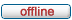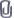# forum.alglib.net

ALGLIB forum
 It is currently Mon May 29, 2023 5:51 am

 All times are UTC

### Forum rules

1. This forum can be used for discussion of both ALGLIB-related and general numerical analysis questions
2. This forum is English-only - postings in other languages will be removed.

 Print view Previous topic | Next topic
Author Message
 Post subject: Double Exponential curve fittingPosted: Wed Mar 20, 2013 1:45 pmJoined: Wed Mar 20, 2013 11:21 am
Posts: 4
Hi there:

I am trying to solve a complex problem.

I have a Matrix with M values inside. Let?s call it: Comptes.

Matrix:
Channel Comptes
700 6
701 20
702 5
. .
. .
. .
800 7

M goes from 700 to 820. (length 120)

Well, I need that Summation from c = 700 to 820 of (Comptes_c - Comptes_Gauss_c)^2 = min

Where Comptes_Gauss = A*exp(-(c-Xk)^2/(2s^2))+B*exp(-(c-XL)^2/(2s^2))

(c = Channel)
s = sigma is a constant: 6.3

So I have to find the best suitable values for A, B, Xk and XL in order to minimize the given Summation.
I have initial approximate values to start using Min L/M iteration:
A=250
Xk=750
B=200
XL=775

I have tried to code this:

Code:
Memo1.Clear;
//We have 4 var: A, Xk, B, XLa
N := 4;

SetLength(S, N);

//S: Our initial guess
S:=250; S:=750; S:=200; S:=775;

M := 120;
SetLength(X1, M);
SetLength(Y1, M);
I:=0;
while I<=M-1 do
begin
X1[I] := 700+I;
//We read the 120 values from a memo (mValores)
Y1[I] := strtofloat(mValores.Lines[i]);
Inc(I);
end;

//
// Now S stores starting point, X and Y store points being fitted.
MinLMCreateFJ(N, M, S, State);
MinLMSetCond(State, 0.0, 0.0, 0.001, 0);
while MinLMIteration(State) do
begin

if State.NeedF then
begin
State.F := 0;
end;
I:=0;
while I<=M-1 do
begin

//
// "A" is stored in State.X
// "Xk" - State.X
// "B" - State.X
// "XLa" - State.X
//

FI := Y1[I]-State.X*exp((-1)*((AP_Sqr(X1[I]-State.X)/(2*AP_Sqr(6.3)))))-State.X*exp((-1)*((AP_Sqr(X1[I]-State.X)/(2*AP_Sqr(6.3)))));

if State.NeedF then
begin
//Summation here?
State.F := State.F+AP_Sqr(FI);
end;
if State.NeedFiJ then
begin
//
// Fi
//
State.Fi[I] := FI;

//
// dFi/dA = -exp^(-((x-Xk)^2)/2s^2)
//
State.J[I,0] := (-1)*exp((-1)*((AP_Sqr(X1[I]-State.X)/(2*AP_Sqr(6.3)))));
//
// dFi/dXk = (A*(xK-x)*exp(-(((x-Xk)^2)/(2*s^2))))))/(6.3^2))

//
State.J[I,1] := (State.X*(State.X-X1[I])*exp((-1)*((AP_Sqr(X1[I]-State.X)/(2*AP_Sqr(6.3))))))/(AP_Sqr(6.3));

//
// dFi/dB = -exp^(-((x-XL)^2)/2s^2)
//
State.J[I,2] := (-1)*exp((-1)*((AP_Sqr(X1[I]-State.X)/(2*AP_Sqr(6.3)))));

//
// dFi/dXLa = (B*(xL-x)*exp(-(((x-xL)^2)/(2*s^2))))))/(6.3^2))
//
State.J[I,3] := (State.X*(State.X-X1[I])*exp((-1)*((AP_Sqr(X1[I]-State.X)/(2*AP_Sqr(6.3))))))/(AP_Sqr(6.3));

end;
Inc(I);
end;
end;
MinLMResults(State, S, Rep);

//
// output results
//
S]));
S]));
S]));
S]));
Memo1.Lines.Add(Format('TerminationType = %0d (should be 2 - stopping when step is small enough)'#13#10'',[
Rep.TerminationType]));

Excel does a pretty good job with Solver and find the next values:
A=144,58
Xk=755,95
B=278,40
XL=772,68

I have uploaded the excel file I am using where you can see the real values and the used ecuations in a more graphic way.

However, when I use the Delphi implementation, I get wrong values for A, Xk, B, and XL. I don?t know what is wrong (maybe derivatives?)

 Attachments: File comment: Excel file with text values and graphic ecuationsChannel_Values.xlsx [15.23 KiB] Downloaded 317 times
TopPost subject: Re: Double Exponential curve fittingPosted: Thu Mar 21, 2013 6:24 pmJoined: Wed Mar 20, 2013 11:21 am
Posts: 4
A partner has already found the mistake:

Code:
...   ))-State.X*exp((-1)*((AP_S ...

Instead of "State.X", it should have been "State.X"

Thanks anyway!

TopDisplay posts from previous: All posts1 day7 days2 weeks1 month3 months6 months1 year Sort by AuthorPost timeSubject AscendingDescending

 All times are UTC

#### Who is online

Users browsing this forum: No registered users and 6 guests

 You cannot post new topics in this forumYou cannot reply to topics in this forumYou cannot edit your posts in this forumYou cannot delete your posts in this forumYou cannot post attachments in this forum

Search for: